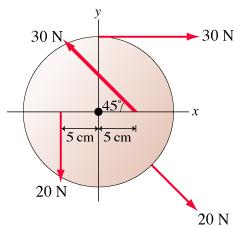# Problem: The 20-cm-diameter disk in the figure can rotate on an axle through its center.What is the net torque about the axle?

###### FREE Expert Solution

Torque:

$\overline{)\begin{array}{rcl}{\mathbf{\tau }}& {\mathbf{=}}& \mathbf{r}\mathbf{×}\mathbf{F}\\ & {\mathbf{=}}& \mathbf{r}\mathbf{F}\mathbf{s}\mathbf{i}\mathbf{n}\mathbf{\theta }\end{array}}$

r = 20/2 = 10 cm

The torque about the axle is given by:

95% (29 ratings)###### Problem DetailsThe 20-cm-diameter disk in the figure can rotate on an axle through its center.

What is the net torque about the axle?